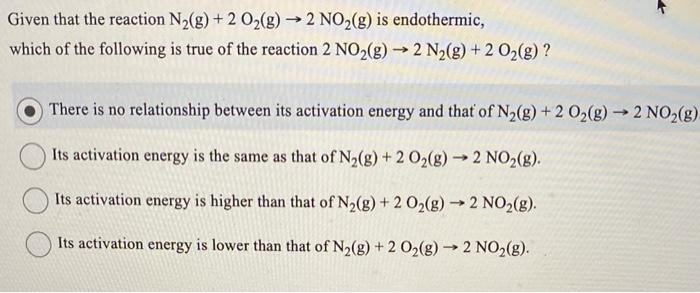Home / Expert Answers / Chemistry / given-that-the-reaction-mathrm-n-2-mathrm-g-2-mathrm-o-2-mathrm-g-rightarrow-2-pa550

# (Solved): Given that the reaction $$\mathrm{N}_{2}(\mathrm{~g})+2 \mathrm{O}_{2}(\mathrm{~g}) \rightarrow 2 ...Given that the reaction \( \mathrm{N}_{2}(\mathrm{~g})+2 \mathrm{O}_{2}(\mathrm{~g}) \rightarrow 2 \mathrm{NO}_{2}(\mathrm{~g})$$ is endothermic, which of the following is true of the reaction $$2 \mathrm{NO}_{2}(\mathrm{~g}) \rightarrow 2 \mathrm{~N}_{2}(\mathrm{~g})+2 \mathrm{O}_{2}(\mathrm{~g})$$ ? There is no relationship between its activation energy and that of $$\mathrm{N}_{2}(\mathrm{~g})+2 \mathrm{O}_{2}(\mathrm{~g}) \rightarrow 2 \mathrm{NO}_{2}(\mathrm{~g})$$ Its activation energy is the same as that of $$\mathrm{N}_{2}(\mathrm{~g})+2 \mathrm{O}_{2}(\mathrm{~g}) \rightarrow 2 \mathrm{NO}_{2}(\mathrm{~g})$$. Its activation energy is higher than that of $$\mathrm{N}_{2}(\mathrm{~g})+2 \mathrm{O}_{2}(\mathrm{~g}) \rightarrow 2 \mathrm{NO}_{2}(\mathrm{~g})$$. Its activation energy is lower than that of $$\mathrm{N}_{2}(\mathrm{~g})+2 \mathrm{O}_{2}(\mathrm{~g}) \rightarrow 2 \mathrm{NO}_{2}(\mathrm{~g})$$.

We have an Answer from Expert